# vol_stability_slides.ppt

2 Jun 2023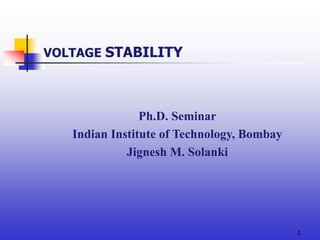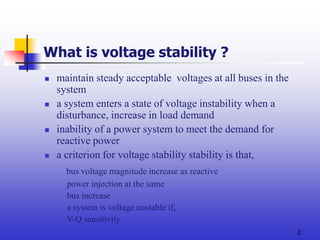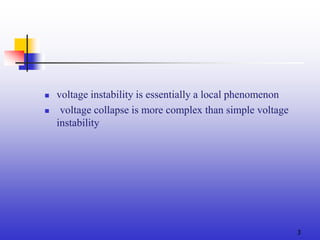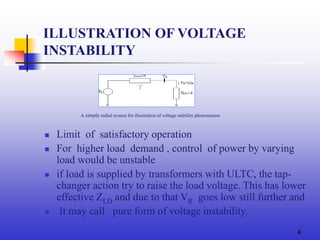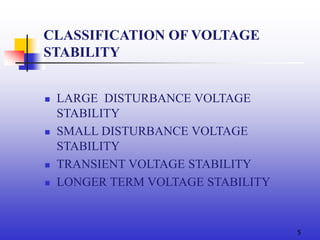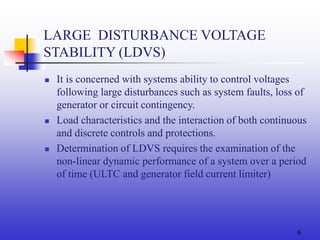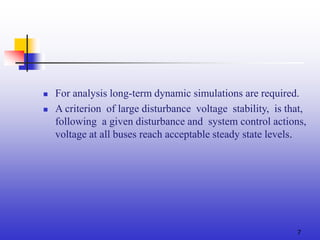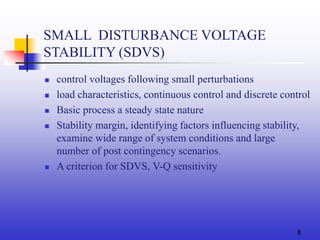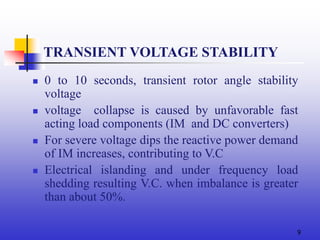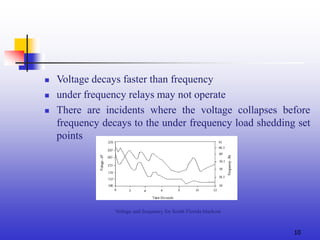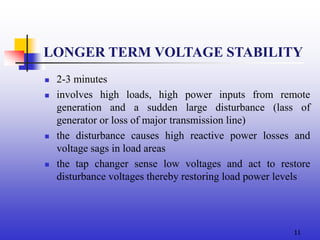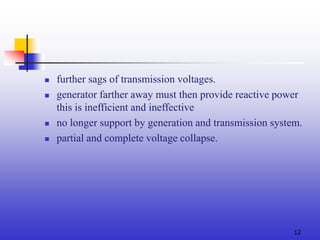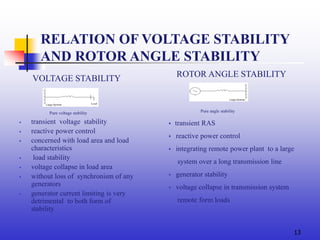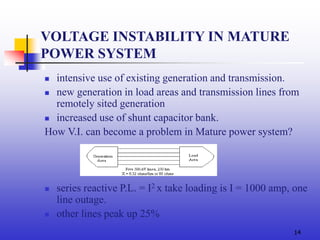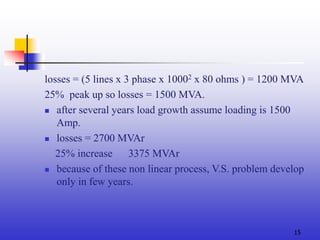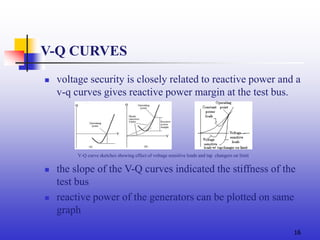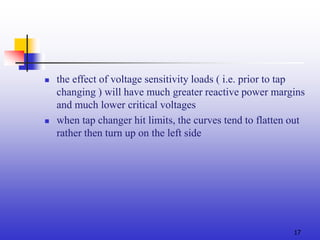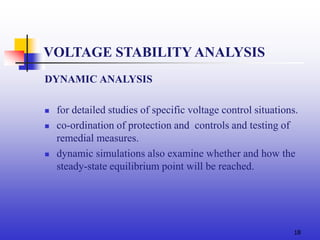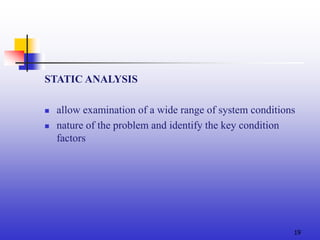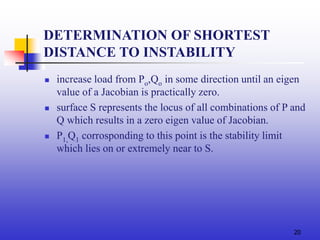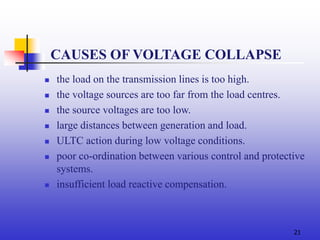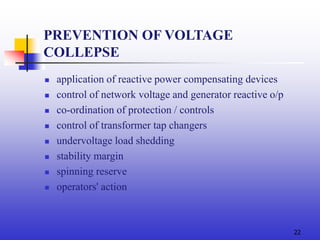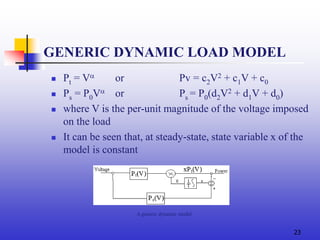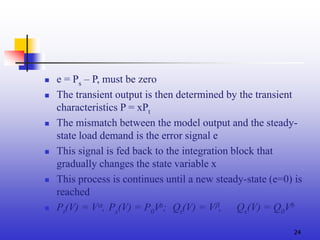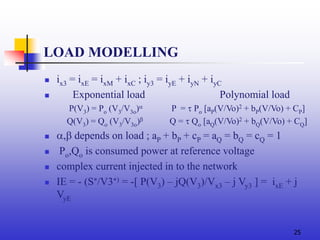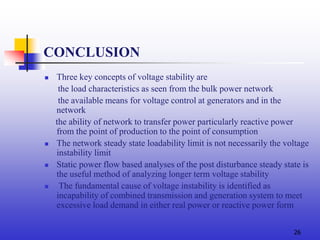1 sur 28

### vol_stability_slides.ppt

• 1. 1 VOLTAGE STABILITY Ph.D. Seminar Indian Institute of Technology, Bombay Jignesh M. Solanki
• 2. 2 What is voltage stability ?  maintain steady acceptable voltages at all buses in the system  a system enters a state of voltage instability when a disturbance, increase in load demand  inability of a power system to meet the demand for reactive power  a criterion for voltage stability stability is that, bus voltage magnitude increase as reactive power injection at the same bus increase a system is voltage unstable if, V-Q sensitivity
• 3. 3  voltage instability is essentially a local phenomenon  voltage collapse is more complex than simple voltage instability
• 4. 4 ILLUSTRATION OF VOLTAGE INSTABILITY  Limit of satisfactory operation  For higher load demand , control of power by varying load would be unstable  if load is supplied by transformers with ULTC, the tap- changer action try to raise the load voltage. This has lower effective ZLD and due to that VR goes low still further and  It may call pure form of voltage instability. A simple radial system for illustration of voltage stability phenomenon
• 5. 5 CLASSIFICATION OF VOLTAGE STABILITY  LARGE DISTURBANCE VOLTAGE STABILITY  SMALL DISTURBANCE VOLTAGE STABILITY  TRANSIENT VOLTAGE STABILITY  LONGER TERM VOLTAGE STABILITY
• 6. 6 LARGE DISTURBANCE VOLTAGE STABILITY (LDVS)  It is concerned with systems ability to control voltages following large disturbances such as system faults, loss of generator or circuit contingency.  Load characteristics and the interaction of both continuous and discrete controls and protections.  Determination of LDVS requires the examination of the non-linear dynamic performance of a system over a period of time (ULTC and generator field current limiter)
• 7. 7  For analysis long-term dynamic simulations are required.  A criterion of large disturbance voltage stability, is that, following a given disturbance and system control actions, voltage at all buses reach acceptable steady state levels.
• 8. 8  control voltages following small perturbations  load characteristics, continuous control and discrete control  Basic process a steady state nature  Stability margin, identifying factors influencing stability, examine wide range of system conditions and large number of post contingency scenarios.  A criterion for SDVS, V-Q sensitivity SMALL DISTURBANCE VOLTAGE STABILITY (SDVS)
• 9. 9 TRANSIENT VOLTAGE STABILITY  0 to 10 seconds, transient rotor angle stability voltage  voltage collapse is caused by unfavorable fast acting load components (IM and DC converters)  For severe voltage dips the reactive power demand of IM increases, contributing to V.C  Electrical islanding and under frequency load shedding resulting V.C. when imbalance is greater than about 50%.
• 10. 10  Voltage decays faster than frequency  under frequency relays may not operate  There are incidents where the voltage collapses before frequency decays to the under frequency load shedding set points Voltage and frequency for South Florida blackout
• 11. 11 LONGER TERM VOLTAGE STABILITY  2-3 minutes  involves high loads, high power inputs from remote generation and a sudden large disturbance (lass of generator or loss of major transmission line)  the disturbance causes high reactive power losses and voltage sags in load areas  the tap changer sense low voltages and act to restore disturbance voltages thereby restoring load power levels
• 12. 12  further sags of transmission voltages.  generator farther away must then provide reactive power this is inefficient and ineffective  no longer support by generation and transmission system.  partial and complete voltage collapse.
• 13. 13 RELATION OF VOLTAGE STABILITY AND ROTOR ANGLE STABILITY VOLTAGE STABILITY Pure voltage stability  transient voltage stability  reactive power control  concerned with load area and load characteristics  load stability  voltage collapse in load area  without loss of synchronism of any generators  generator current limiting is very detrimental to both form of stability ROTOR ANGLE STABILITY Pure angle stability  transient RAS  reactive power control  integrating remote power plant to a large system over a long transmission line  generator stability  voltage collapse in transmission system remote form loads
• 14. 14 VOLTAGE INSTABILITY IN MATURE POWER SYSTEM  intensive use of existing generation and transmission.  new generation in load areas and transmission lines from remotely sited generation  increased use of shunt capacitor bank. How V.I. can become a problem in Mature power system?  series reactive P.L. = I2 x take loading is I = 1000 amp, one line outage.  other lines peak up 25%
• 15. 15 losses = (5 lines x 3 phase x 10002 x 80 ohms ) = 1200 MVA 25% peak up so losses = 1500 MVA.  after several years load growth assume loading is 1500 Amp.  losses = 2700 MVAr 25% increase 3375 MVAr  because of these non linear process, V.S. problem develop only in few years.
• 16. 16 V-Q CURVES  voltage security is closely related to reactive power and a v-q curves gives reactive power margin at the test bus.  the slope of the V-Q curves indicated the stiffness of the test bus  reactive power of the generators can be plotted on same graph V-Q curve sketches showing effect of voltage sensitive loads and tap changers on limit
• 17. 17  the effect of voltage sensitivity loads ( i.e. prior to tap changing ) will have much greater reactive power margins and much lower critical voltages  when tap changer hit limits, the curves tend to flatten out rather then turn up on the left side
• 18. 18 VOLTAGE STABILITY ANALYSIS DYNAMIC ANALYSIS  for detailed studies of specific voltage control situations.  co-ordination of protection and controls and testing of remedial measures.  dynamic simulations also examine whether and how the steady-state equilibrium point will be reached.
• 19. 19 STATIC ANALYSIS  allow examination of a wide range of system conditions  nature of the problem and identify the key condition factors
• 20. 20 DETERMINATION OF SHORTEST DISTANCE TO INSTABILITY  increase load from Po,Qo in some direction until an eigen value of a Jacobian is practically zero.  surface S represents the locus of all combinations of P and Q which results in a zero eigen value of Jacobian.  P1,Q1 corrosponding to this point is the stability limit which lies on or extremely near to S.
• 21. 21  the load on the transmission lines is too high.  the voltage sources are too far from the load centres.  the source voltages are too low.  large distances between generation and load.  ULTC action during low voltage conditions.  poor co-ordination between various control and protective systems.  insufficient load reactive compensation. CAUSES OF VOLTAGE COLLAPSE
• 22. 22 PREVENTION OF VOLTAGE COLLEPSE  application of reactive power compensating devices  control of network voltage and generator reactive o/p  co-ordination of protection / controls  control of transformer tap changers  undervoltage load shedding  stability margin  spinning reserve  operators' action
• 23. 23 GENERIC DYNAMIC LOAD MODEL  Pt = V or Pv = c2V2 + c1V + c0  Ps = P0V or Ps = P0(d2V2 + d1V + d0)  where V is the per-unit magnitude of the voltage imposed on the load  It can be seen that, at steady-state, state variable x of the model is constant A generic dynamic model
• 24. 24  e = Ps – P, must be zero  The transient output is then determined by the transient characteristics P = xPt  The mismatch between the model output and the steady- state load demand is the error signal e  This signal is fed back to the integration block that gradually changes the state variable x  This process is continues until a new steady-state (e=0) is reached  Pt(V) = V, Ps(V) = P0Va; Qt(V) = V, Qs(V) = Q0Vb
• 25. 25 LOAD MODELLING  ix3 = ixE = ixM + ixC ; iy3 = iyE + iyN + iyC  Exponential load Polynomial load P(V3) = Po (V3/V3o) P =  Po [aP(V/Vo)2 + bP(V/Vo) + CP] Q(V3) = Qo (V3/V3o) Q =  Qo [aQ(V/Vo)2 + bQ(V/Vo) + CQ]  , depends on load ; aP + bP + cP = aQ = bQ = cQ = 1  Po,Qo is consumed power at reference voltage  complex current injected in to the network  IE = - (S/V3) = -[ P(V3) – jQ(V3)/Vx3 – j Vy3 ] = ixE + j VyE
• 26. 26 CONCLUSION  Three key concepts of voltage stability are the load characteristics as seen from the bulk power network the available means for voltage control at generators and in the network the ability of network to transfer power particularly reactive power from the point of production to the point of consumption  The network steady state loadability limit is not necessarily the voltage instability limit  Static power flow based analyses of the post disturbance steady state is the useful method of analyzing longer term voltage stability  The fundamental cause of voltage instability is identified as incapability of combined transmission and generation system to meet excessive load demand in either real power or reactive power form
• 28. 28